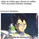5523 visningar
Filled support resistance zones for better visualization.
```//Created by CristianD
study(title="CD DeMark Range Expansion V1", shorttitle="CD DeMark Range Expansion V1", overlay=true)
sr = input(true, title="Show Daily Range")

range = high - low

// Daily line breaks
sopen = security(tickerid, "D", open)

a=range*0.382
b=range*0.618
h=sopen+a
h1=sopen+b
l=sopen-a
l1=sopen-b

// Color
hcolor=sopen != sopen ? na : maroon
hcolor1=sopen != sopen ? na : red
lcolor=sopen != sopen ? na : green
lcolor1=sopen != sopen ? na : lime

//Daily Range
highrange1 = security(tickerid, 'D', h)
highrange2 = security(tickerid, 'D', h1)
lowrange1 = security(tickerid, 'D', l)
lowrange2 = security(tickerid, 'D', l1)

offs_daily = 0
H1=plot(sr and highrange1 ? highrange1 : na, title="Daily High1",color=hcolor, linewidth=1)
H2=plot(sr and highrange2 ? highrange2 : na, title="Daily Daily High2", color=hcolor1, linewidth=1)
L1=plot(sr and lowrange1 ? lowrange1 : na, title="Daily Low1",color=lcolor, linewidth=1)
L2=plot(sr and lowrange2 ? lowrange2 : na, title="Daily Daily Low2", color=lcolor1, linewidth=1)

fill(H1,H2,color=lime)
fill(L1,L2,color=maroon)

```

## Kommentarerwhats the difference between ADR and this indictor ?
Svara